﻿ 节制闸与泵站联合工作的数值模拟研究
«上一篇文章快速检索 高级检索

 哈尔滨工程大学学报2019, Vol. 40Issue (4): 663-669  DOI: 10.11990/jheu.2017090520

### 引用本文LI Gang, LUO Ming, GENG Jing, et al. Numerical simulation of combined work of check gate and pumping station[J]. Journal of Harbin Engineering University, 2019, 40(4), 663-669. DOI: 10.11990/jheu.201709052.### 文章历史

1. 哈尔滨工程大学 船舶工程学院, 黑龙江 哈尔滨 150001;
2. 四川大学 水力学与山区河流开发保护国家重点实验室, 四川 成都 610065

Numerical simulation of combined work of check gate and pumping station
LI Gang 1, LUO Ming 2, GENG Jing 1, MA Shiling 1
1. College of Shipbuilding Engineering, Harbin Engineering University, Harbin 150001, China;
2. State Key Laboratory of Hydraulics and Mountain River Engineering, Sichuan University, Chengdu 610065, China
Abstract: It is difficult to determine the flow field affected by the combined work of the check gate and pumping station.Numerical simulation of combined work of a check gate and pumping station in the channel of the Qinglongshan irrigation district was conducted by using MIKE21 FM hydrodynamic model.Detailed water surface and flow field data of the check gate and pumping station were obtained, and the influence between the check gate and pumping station was analyzed.Results show that the flow field of the check gate is asymmetrical and certain problems occur, such as flow velocity concentration and flow rate exceeding the scope of the revetment, which is affected by the pumping station.Four optimized layout of check gate and pumping station are proposed to address these problems.These optimization schemes are validated by numerical simulation by flow asymmetry and stability, which provides an important reference for the arrangement of the combined work of the check gate and pumping station.
Keywords: MIKE21    hydrodynamic model    check gate    pumping station    combined work    numerical simulation    flow field analysis    optimization

1 青龙山灌区数学模型

1.1 控制方程

Mike21 FM水动力模型是基于三向不可压缩和Reynolds值均布的Navier-Stokes方程，并服从于Boussinesq假定和静水压力的假定，模型考虑了科氏力、涡黏性等方面[11-14]

 $\frac{{\partial h}}{{\partial t}} + \frac{{\partial h\bar u}}{{\partial x}} + \frac{{\partial h\bar v}}{{\partial y}} = hS$ (1)

X方向水平动量方程为：

 $\begin{array}{l} \frac{{\partial h\bar u}}{{\partial t}} + \frac{{\partial h\;{{\bar u}^2}}}{{\partial x}} + \frac{{\partial h\;\bar u\bar v}}{{\partial y}} = f\bar vh - gh\frac{{\partial \eta }}{{\partial x}} - \\ \frac{h}{{{\rho _0}}}\frac{{\partial {p_a}}}{{\partial x}} - \frac{{g{h^2}}}{{2{\rho _0}}}\frac{{\partial \rho }}{{\partial x}} + \frac{{{\tau _{sx}}}}{{{\rho _0}}} - \frac{{{\tau _{bx}}}}{{{\rho _0}}} - \frac{1}{{{\rho _0}}}\left( {\frac{{\partial {s_{xx}}}}{{\partial x}} + \frac{{\partial {s_{xy}}}}{{\partial y}}} \right) + \\ \frac{\partial }{{\partial x}}\left( {h{T_{xx}}} \right) + \frac{\partial }{{\partial y}}\left( {h{T_{xy}}} \right) + h{u_s}S \end{array}$ (2)

Y方向水平动量方程为：

 $\begin{array}{l} \frac{{\partial h\bar v}}{{\partial t}} + \frac{{\partial h\;\bar u\bar v}}{{\partial x}} + \frac{{\partial h\;{{\bar v}^2}}}{{\partial y}} = - f\bar uh - gh\frac{{\partial \eta }}{{\partial y}} - \\ \frac{h}{{{\rho _0}}}\frac{{\partial {p_a}}}{{\partial y}} - \frac{{g{h^2}}}{{2{\rho _0}}}\frac{{\partial \rho }}{{\partial y}} + \frac{{{\tau _{sy}}}}{{{\rho _0}}} - \frac{{{\tau _{by}}}}{{{\rho _0}}} - \frac{1}{{{\rho _0}}}\left( {\frac{{\partial {s_{yx}}}}{{\partial x}} + \frac{{\partial {s_{yy}}}}{{\partial y}}} \right) + \\ \frac{\partial }{{\partial x}}\left( {h{T_{xy}}} \right) + \frac{\partial }{{\partial y}}\left( {h{T_{yy}}} \right) + h{v_s}S \end{array}$ (3)

1.2 边界条件和网格划分 1.2.1 模拟工况的确定Download: 图 1 青龙山灌区 Fig. 1 Qinglong irrigation district

1.2.2 几何模型和网格划分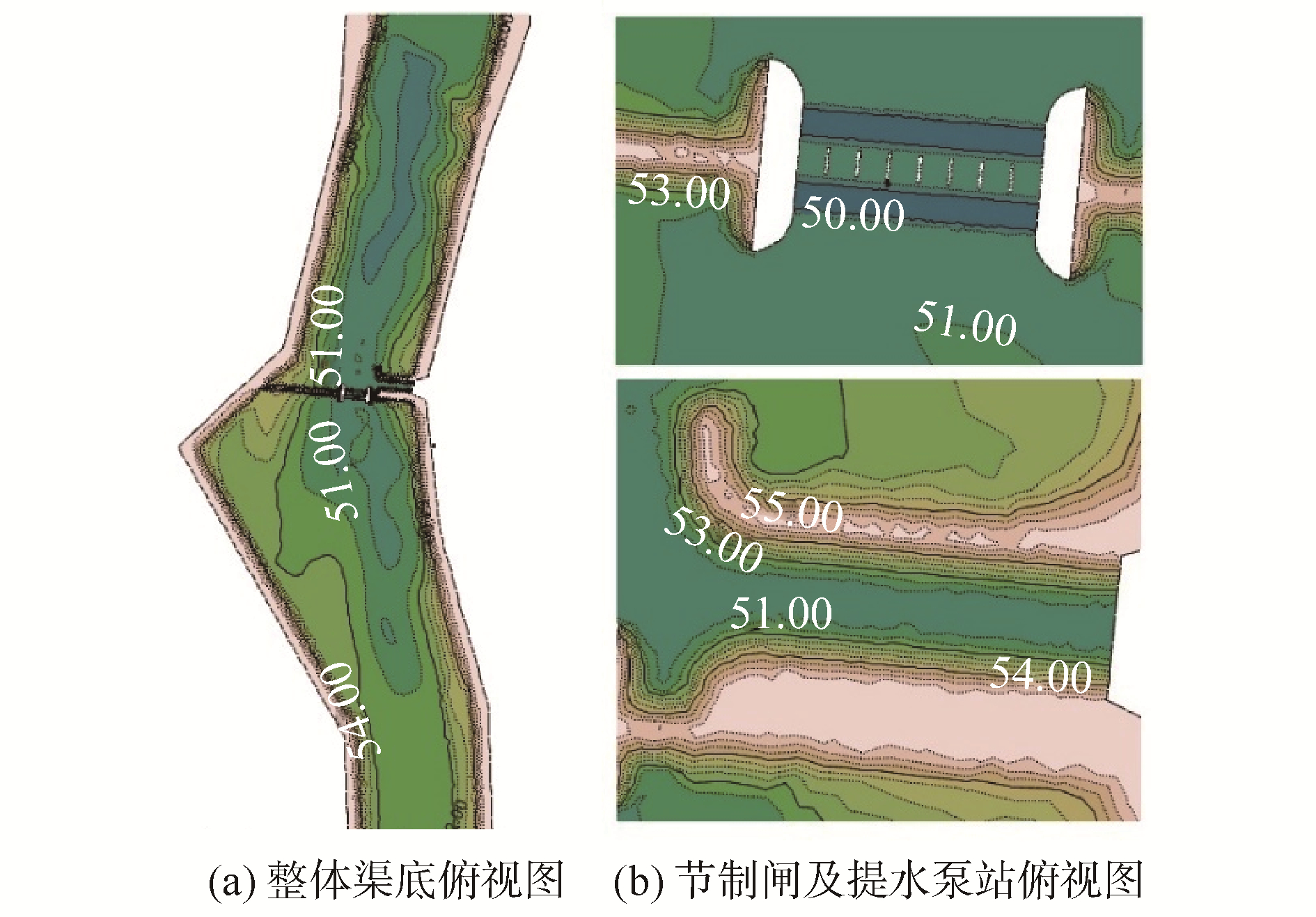Download: 图 2 几何模型 Fig. 2 The geometric model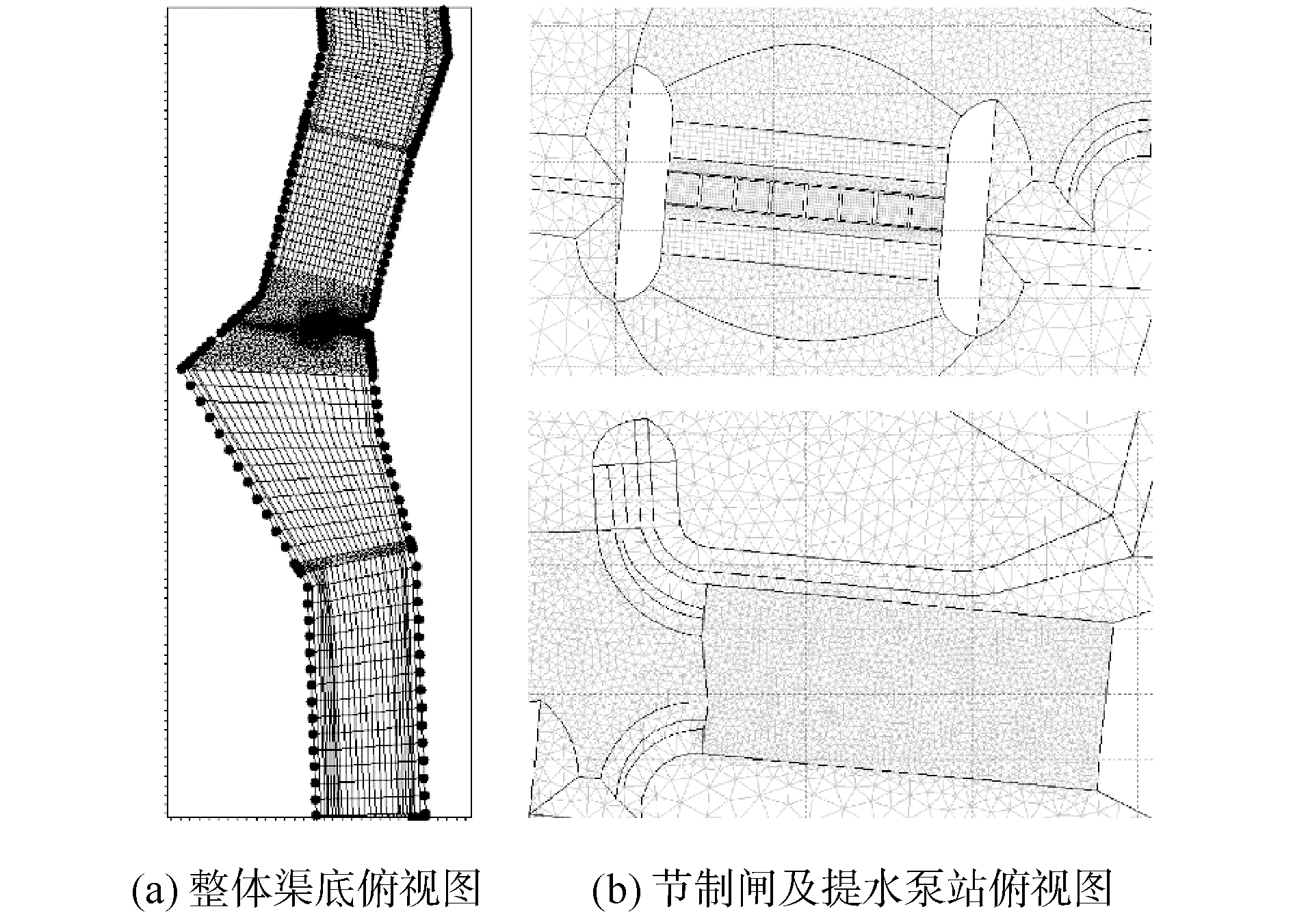Download: 图 3 网格划分 Fig. 3 Simulated mesh map
1.2.3 边界条件和计算控制条件表 1 模拟工况边界条件表 Table 1 Boundary conditions of simulation mode

2 数值模型的率定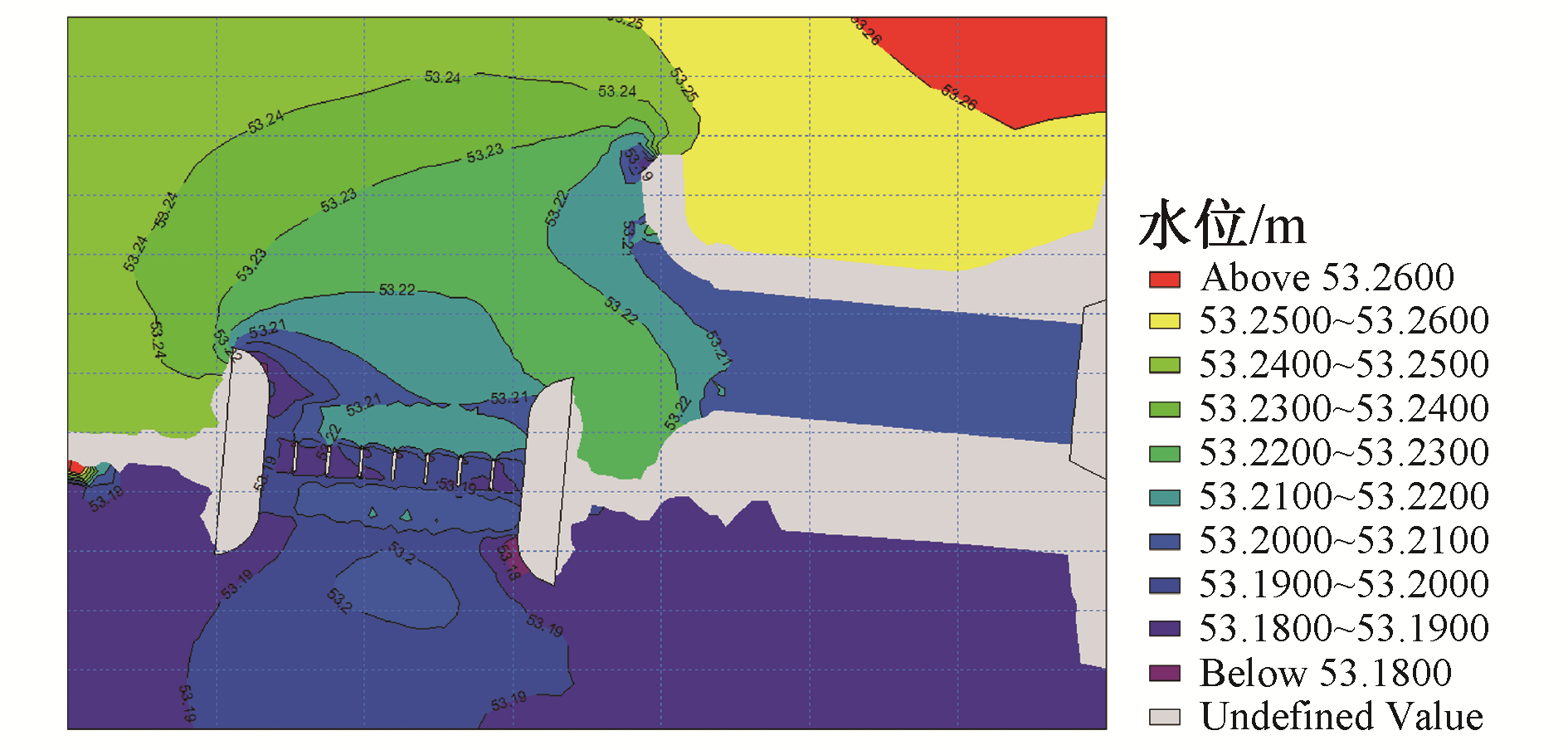Download: 图 5 一期设计水位等值线 Fig. 5 Water level contour map of the first design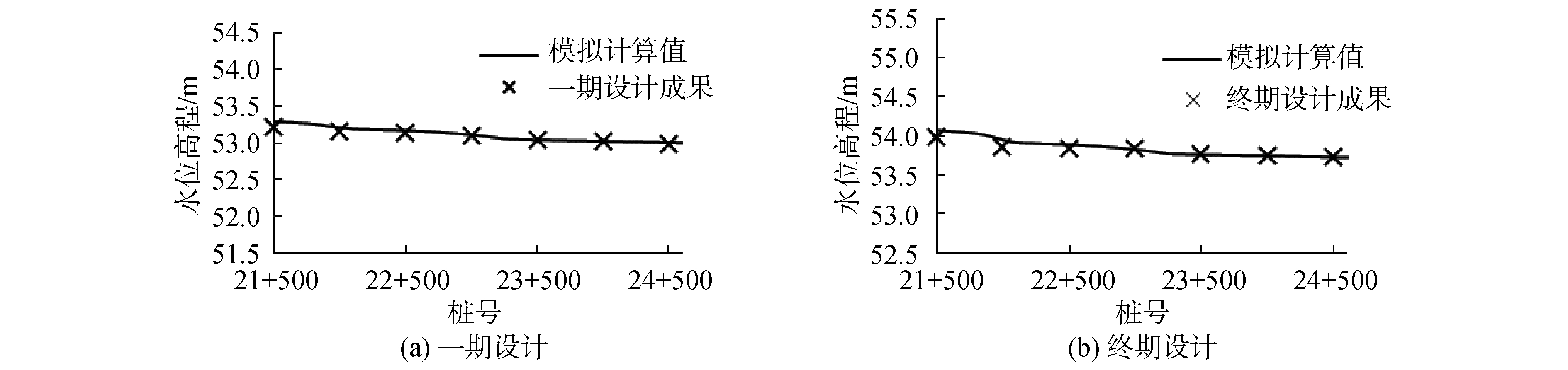Download: 图 4 纵向水面线对比 Fig. 4 Comparison of longitudinal water surface profile
3 模拟结果及分析 3.1 典型断面与坐标的确定

3.2 一期设计模拟结果 3.2.1 水面线分析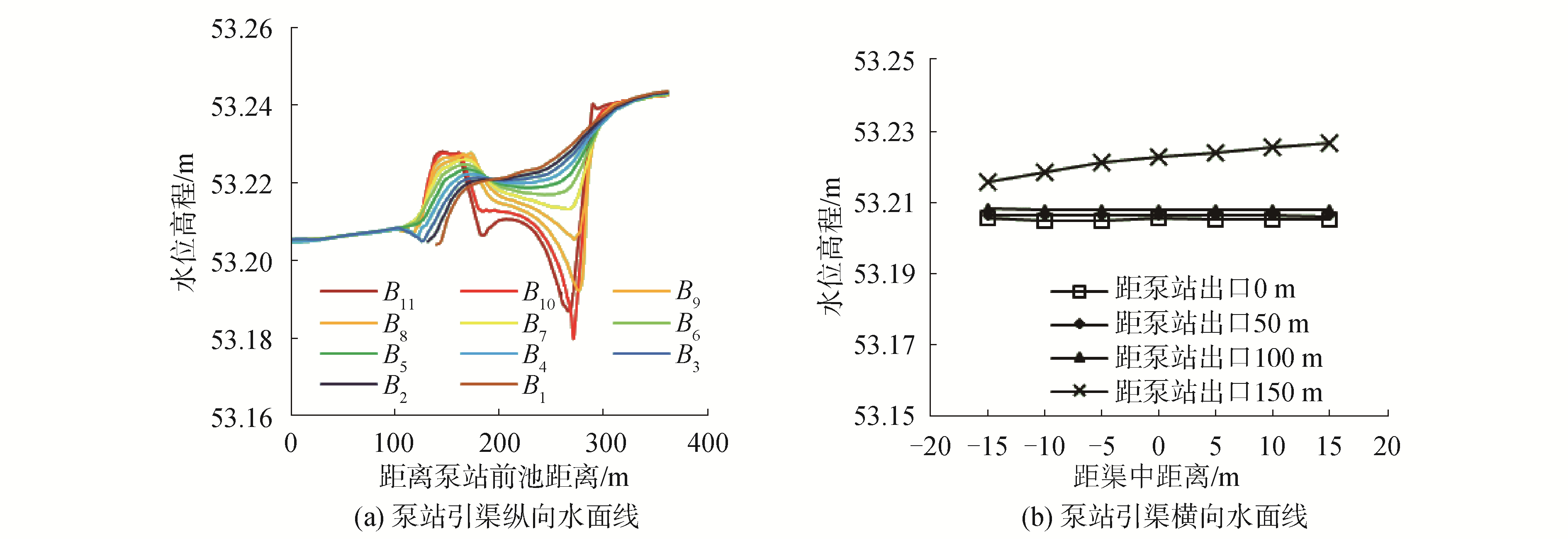Download: 图 6 一期设计水面线 Fig. 6 The longitudinal water surface line of the first design
3.2.2 流场分析Download: 图 7 一期设计泵站及1#节制闸流场图 Fig. 7 The flow field diagram of the Pumping Station and 1# check gate e of the first design

3.3 终期设计模拟结果 3.3.1 水面线分析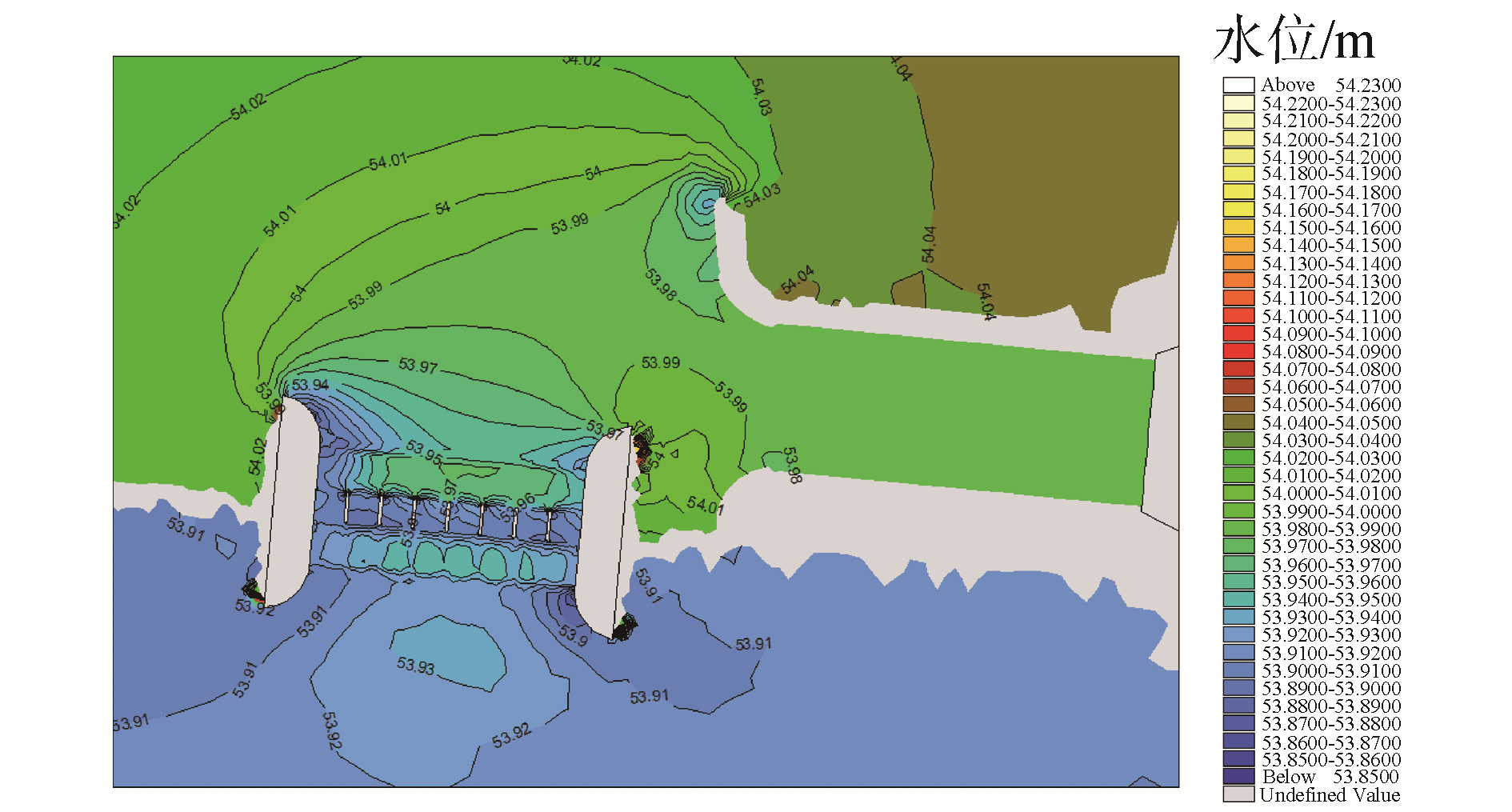Download: 图 8 终期设计水位等值线 Fig. 8 Water level contour map of the last design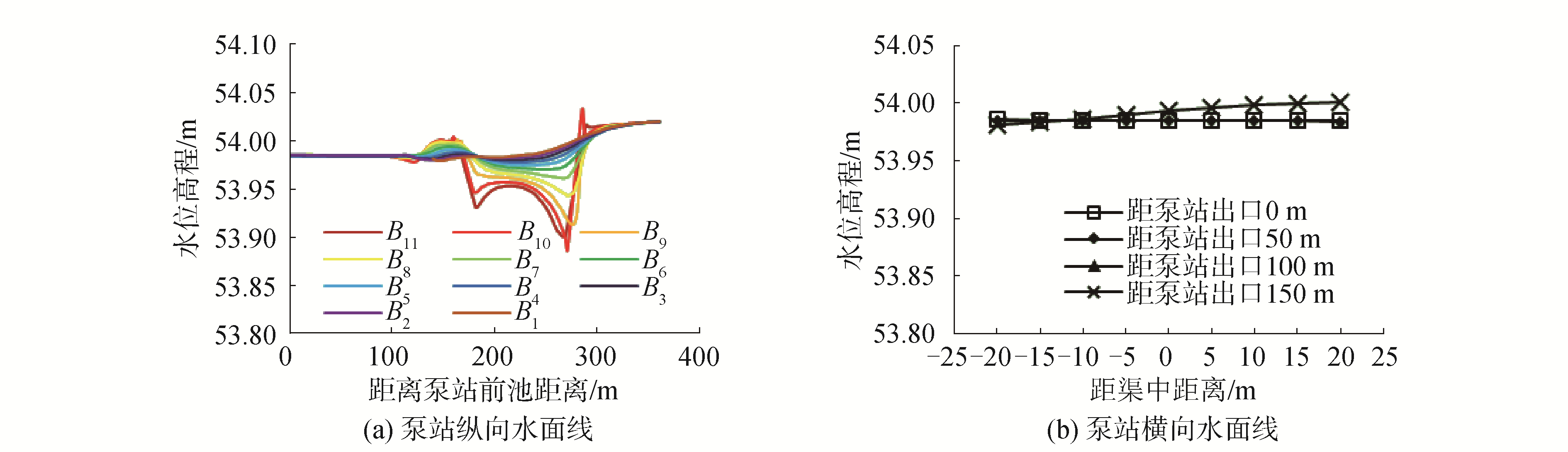Download: 图 9 终期设计水面线 Fig. 9 The longitudinal water surface line of the last designDownload: 图 10 泵站及1#节制闸流场图 Fig. 10 The flow field diagram of the Pumping Station and 1# check gate of the last design
3.3.2 流场分析

3.4 综合分析

4 优化方案及流场分析 4.1 优化方案的确定表 2 优化方案 Table 2 Optimization schemes
4.2 优化方案模拟结果及分析 4.2.1 水面线分析Download: 图 11 优化方案水位等值线图 Fig. 11 The water-level contour line map of optimization schemes
4.2.2 流场分析

5 结论

1) 结合MIKE21 FM水动力模块对泵站和节制闸联合工作情况下的流场进行了数值模拟，分析了水面线和流场数据，获得了泵站和节制闸联合工作的相互影响关系。

2) 针对泵站和1#节制闸流场不对称及流速过大的问题，提出了4个优化方案，由各方案模拟结果对比分析，确定方案4对于改善节制闸流动对称性、降低过闸最大流速、降低引渠左导墙处最大流速是最有利的。

  王雷, 史文彪, 杨开林, 等. 南水北调中线惠南庄泵站进水前池布置方案的分析研究[J]. 南水北调与水利科技, 2008, 6(1): 185-193. WANG Lei, SHI Wenbiao, YANG Kailin, et al. Analyses of the layout the forebay of Hui-Nan-Zhuang pump station[J]. South-to-North water transfers and water science and technology, 2008, 6(1): 185-193. DOI:10.3969/j.issn.1672-1683.2008.01.049 (0)  谢省宗, 王雷, 冯宾春, 等. 惠南庄泵站前池流道模型试验研究[J]. 南水北调与水利科技, 2005, 3(4): 4-7. XIE Shengzong, WANG Lei, FENG Binchun, et al. Flow channel hydraulics test for forebay of the Huinanzhuang pumping station[J]. South-to-North water transfers and water science & technology, 2005, 3(4): 4-7. DOI:10.3969/j.issn.1672-1683.2005.04.002 (0)  王芳芳, 吴时强, 肖潇, 等. 三维数值模拟在泵站侧向进水前池的应用[J]. 水利水运工程学报, 2014(2): 54-59. WANG Fangfang, WU Shiqiang, XIAO Xiao, et al. 3-D numerical simulation for side-inlet forebay of a pumping station[J]. Hydro-science and engineering, 2014(2): 54-59. DOI:10.3969/j.issn.1009-640X.2014.02.009 (0)  陈松山, 屈磊飞, 葛强, 等. 泵站枢纽三维湍流数值模拟研究[J]. 扬州大学学报(自然科学版), 2005, 8(2): 70-73. CHEN Songshan, QU Leifei, GE Qiang, et al. Numerical simulation of three-dimension turbulent flow in pumping station projects[J]. Journal of Yangzhou university (natural science edition), 2005, 8(2): 70-73. (0)  高传昌, 刘新阳, 石礼文, 等. 泵站前池与进水池整流方案数值模拟[J]. 水力发电学报, 2011, 30(2): 54-59. GAO Chuanchang, LIU Xinyang, SHI Liwen, et al. Numerical simulation of flow pattern modification schemes for the forebay and suction sump of pumping station[J]. Journal of hydroelectric engineering, 2011, 30(2): 54-59. (0)  虞鸿, 张永进, 刘海泉. 大型泵站前池复杂流场的数值模拟及优化设计[J]. 水电自动化与大坝监测, 2013, 37(4): 66-70. YU Hong, ZHANG Yongjin, LIU Haiquan. Numeric simulation and optimum design for large pump station with complicated flow distribution[J]. Hydropower automation and dam monitoring, 2013, 37(4): 66-70. DOI:10.3969/j.issn.1671-3893.2013.04.017 (0)  CONSTANTINESCU G S, PATEL V C. Numerical model for simulation of pump-intake flow and vortices[J]. Journal of hydraulic engineering, 1998, 124(2): 123-134. DOI:10.1061/(ASCE)0733-9429(1998)124:2(123) (0)  CONSTANTINESCU G S, PATEL V C. Role of turbulence model in prediction of pump-bay vortices[J]. Journal of hydraulic engineering, 2000, 126(5): 387-391. DOI:10.1061/(ASCE)0733-9429(2000)126:5(387) (0)  ALDAMA A A. Pupping rate optimization in a storm drainage system through the combined use of numerical simulation and linear programming[J]. Advances in water resources, 1991, 14(4): 192-202. DOI:10.1016/0309-1708(91)90015-G (0)  ANTONIO P, ALMEIDA S J, ANTONIO A, et al. Socorridos pumping station-numerical modelling[M]//SCHWEIGER H F. Numerical Methods in Geotechnical Engineering: Sixth European Conference on Numerical Methods in Geotechnical Engineering. London: CRC Press, 2006: 367-372. (0)  耿敬, 张洋, 李明伟, 等. 洪水数值模拟的三维动态可视化方法[J]. 哈尔滨工程大学学报, 2018, 39(7): 1179-1185. GENG Jing, ZHANG Yang, LI Mingwei, et al. Research on 3D dynamic visualization based on flood numerical simulation[J]. Journal of Harbin Engineering University, 2018, 39(7): 1179-1185. (0)  CAMASSA R, HOLM D D. An integrable shallow water equation with peaked solitons[J]. Physical review letters, 1993, 71(11): 1661. DOI:10.1103/PhysRevLett.71.1661 (0)  CONSTANTIN A, ESCHER J. Wave breaking for nonlinear nonlocal shallow water equations[J]. Acta mathematica, 1998, 181(2): 229-243. DOI:10.1007/BF02392586 (0)  IVERSON R M. Landslide triggering by rain infiltration[J]. Water resources research, 2000, 36(7): 1897-1910. DOI:10.1029/2000WR900090 (0)  张洪雨, 李葛飞, 李刚.青龙河总干渠灌溉及泄洪能力数模实验研究[R].黑龙江: 黑龙江农垦勘测设计研究院, 2015. (0)